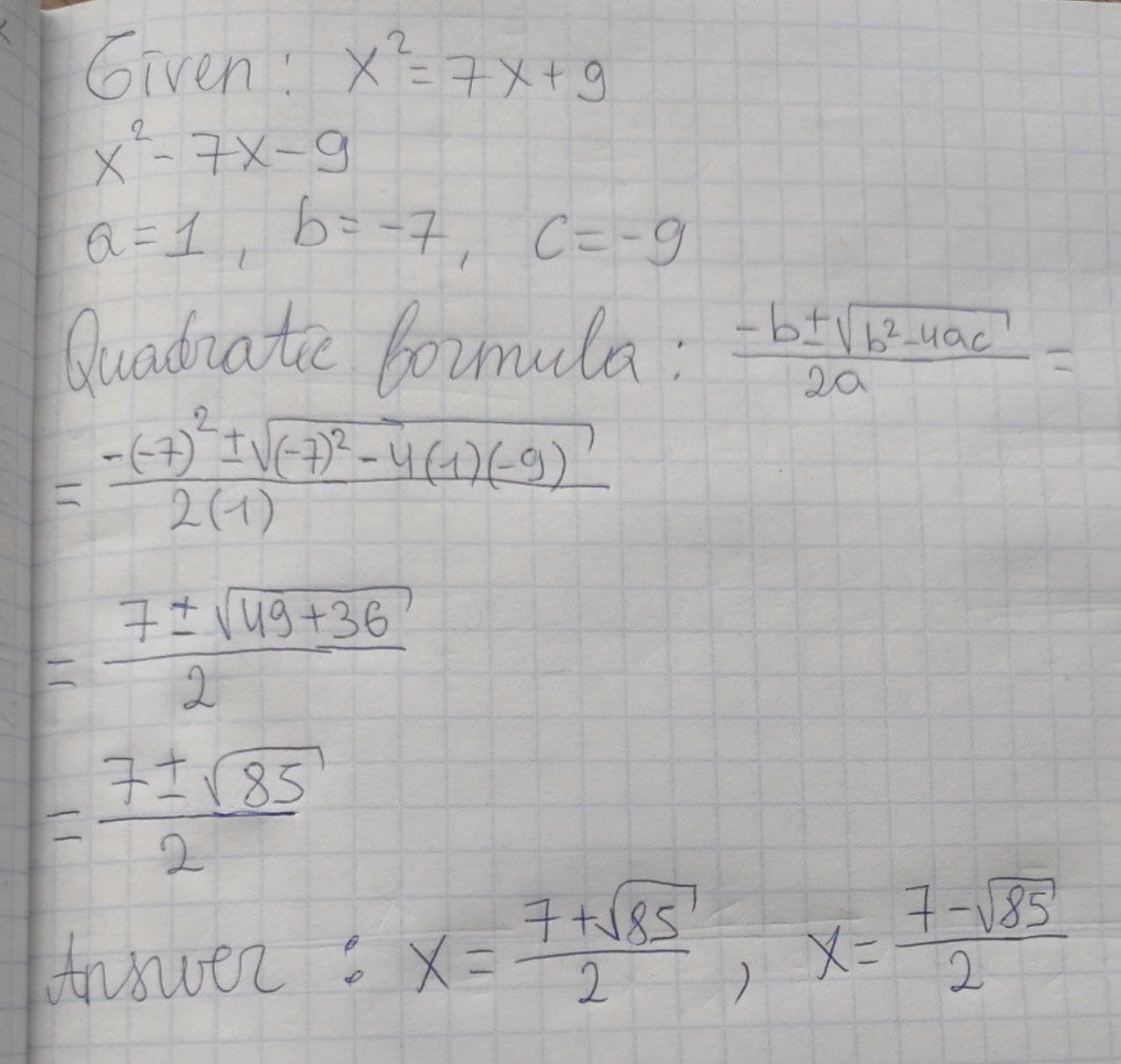# Solve the quadratic by using the quadratic formulax^2 = 7x + 9Lewis Harvey 2021-01-31 Answered

Solve the quadratic by using the quadratic formula ${x}^{2}=7x+9$

You can still ask an expert for help

• Questions are typically answered in as fast as 30 minutes

Solve your problem for the price of one coffee

• Math expert for every subject
• Pay only if we can solve itLeonard Stokes

Quadratic formula: $x=\frac{-b+-\sqrt{{b}^{2}-4ac}}{2a}=\frac{-\left(-7\right)+-\sqrt{\left(-7{\right)}^{2}-4\left(1\right)\left(-9\right)}}{2\left(1\right)}=\frac{7+-\sqrt{49+36}}{2}=\frac{7+-\sqrt{85}}{2}x=\frac{7+\sqrt{85}}{2},\frac{7-\sqrt{85}}{2}$